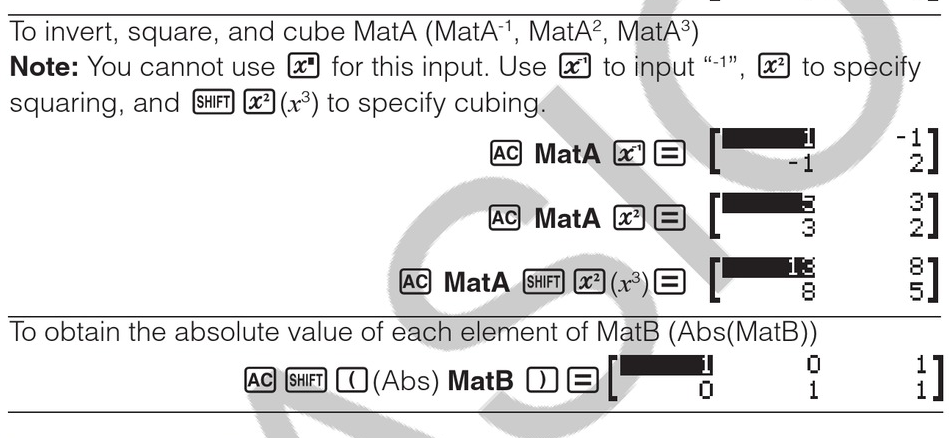Casio fx-991EX Matrix Calculation
02-08-2021, 05:35 AM (This post was last modified: 02-08-2021 06:21 AM by Gamo.)
Post: #1GamoSenior Member Posts: 720 Joined: Dec 2016
Casio fx-991EX Matrix Calculation
Casio fx-991EX manual didn't give example on the use case for the Inverse of the Matrix while

HP-15C User's Handbook gave the example problem for the Inverse Matrix and Multiplication of Matrix to use for the Linear Equation of Unkonws Varibles.

I'll use 15C User's Handbook Example on Page 156 "Title Soving the Equation AX=B"

AX=B equation can be changed to X = A^-1 x B

Example Matrix Equation: On page 157

Here is the steps on how to enter "Matrix Calculations" using Casio fx-991EX with Matrix Mode

[MENU]  // Matrix Mode //
 // Matrix A //
  // Set Matrix A to 2x2 //

 [=]  [=]
[.24] [=] [.86] [=]

[OPTN]   // Define Matrix and Matrix B //
  // Set Matrix B to 2x3

 [=]  [=]  [=]
[120.32] [=] [112.96] [=] [151.36] [=]

[OPTN]  // Matrix Calculation //

[OPTN]  // Seclect Mat A //

Screen Display MatA enter [X^-1] [x] [OPTN] 

Screen Display MatA^-1 x MatB press [=]

MatAns= 186, 141, 215, 88, 92 and 116

Remark:
A system of Linear Equation can be solve by using the [MENU] mode A seclection #1: Simul Equation
for the restriction that constant matrix and solution matrix each had one column.

While the example shown above is for a constant matrix and solution matrix with more than one column.

Gamo 2/2021
03-16-2021, 01:06 AM
Post: #2BINUBALLMember Posts: 133 Joined: Mar 2021
RE: Casio fx-991EX Matrix Calculation
Unfortunately, In 570EX manual 27 page. There are inverse matrix example. but well done!S.Korean / HP-50G | fx-570EX | fx-570CW | HP-200LX
 « Next Oldest | Next Newest »

User(s) browsing this thread: 1 Guest(s)